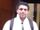## need help for nested case when.

proc sql;
create table check1 as
select *,

case when new=1 then
case when aa=1 then 1 else 0 end as aa1,
case when aa=2 then 1 else 0 end as aa2,
case when aa=3 then 1 else 0 end as aa3

end

from id;
quit;

I wrote the code above, it looks not right.

The question is how I close the big loop for new=1?

thanks

1 ACCEPTED SOLUTION

Accepted Solutionsnovinosrin
Tourmaline | Level 20

## Re: need help for nested case when.

Hi @wwwz  Not quite suited for "nested" case when, rather it's far more convenient and precise to use AND operator like

case when new=1 and  aa=1 then 1 else 0 end as aa1,
case when new=1 and  aa=2 then 1 else 0 end as aa2,
case when new=1 and  aa=3 then 1 else 0 end as aa3

4 REPLIES 4novinosrin
Tourmaline | Level 20

## Re: need help for nested case when.

Hi @wwwz  Not quite suited for "nested" case when, rather it's far more convenient and precise to use AND operator like

case when new=1 and  aa=1 then 1 else 0 end as aa1,
case when new=1 and  aa=2 then 1 else 0 end as aa2,
case when new=1 and  aa=3 then 1 else 0 end as aa3

thanks

## Re: need help for nested case when.

@wwwz wrote:

proc sql;
create table check1 as
select *,

case when new=1 then
case when aa=1 then 1 else 0 end as aa1,
case when aa=2 then 1 else 0 end as aa2,
case when aa=3 then 1 else 0 end as aa3

end

from id;
quit;

I wrote the code above, it looks not right.

The question is how I close the big loop for new=1?

thanks

Might say this is more of a data step task:

```data check1;
set id;
if new=1 then do;
aa1 = (aa=1);
aa2 = (aa=2);
aa3 = (aa=3);
end;
run;```

Especially if you have may more values to check. Consider if AA takes values of 1 to 25. That gets to be a lot of CASE coding.

But with an array in a data step:

```data work.id;
input new aa;
datalines;
1 1
1 3
0 1
1 25
;

data work.check1;
set work.id;
array a(25) aa1-aa25;
if new= 1 then do i= 1 to dim(a);
a[i]= (aa=i);
end;
drop i;
run;```

And ARRAYS do not work in SQL.

## Re: need help for nested case when.

thanks

Discussion stats
• 4 replies
• 988 views
• 0 likes
• 3 in conversation